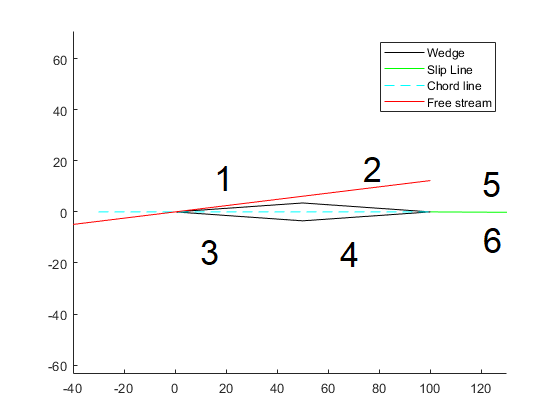## Supersonic symmetric wedge cl and cd calculator function

version 1.0.0 (4.29 KB) by
Given geometry,angle of attack and Mach number, the function calculates the cl, cd and slip line angle of a supersonic symmetric wedge.

Updated 1 Nov 2021

This function calculates the lift and drag coefficients of a symmetric wedge airfoil using Shock-Expansion theory. It also calculates the slip line angle at the trailing edge
The function calls two other functions obliqueshock.m and prandtlmeyer.m also included
P5 and P6 are the values of pressure above and below the slip line respectively just to confirm that the slip line Pressure condition is fulfilled.
• M_entry: Mach number of the flow uptream.
• Ttc: Thickness to chord ratio.
• MaxLoc: Location of the maximum thickness with respect to the chord entered in (ex: MaxLoc=50 is at midchord)
• AOA: Angle of attack the chordline makes with the free stream.
• slip_tol: pressure tolerance above and below the slip line in Pa.
the station numbers over the wedge are as followsThe function works well for undergraduate studies but not suitable for post-graduate work.

### Cite As

Mohamed Hassan (2022). Supersonic symmetric wedge cl and cd calculator function (https://www.mathworks.com/matlabcentral/fileexchange/101390-supersonic-symmetric-wedge-cl-and-cd-calculator-function), MATLAB Central File Exchange. Retrieved .

##### MATLAB Release Compatibility
Created with R2018b
Compatible with any release
##### Platform Compatibility
Windows macOS Linux